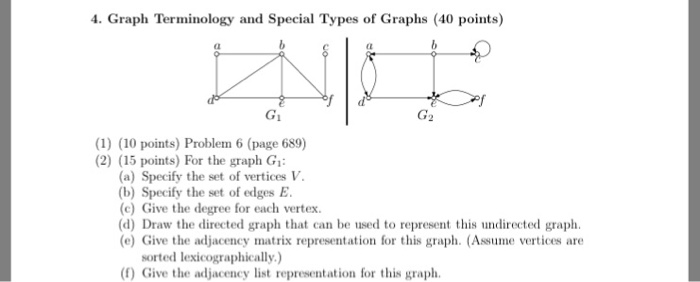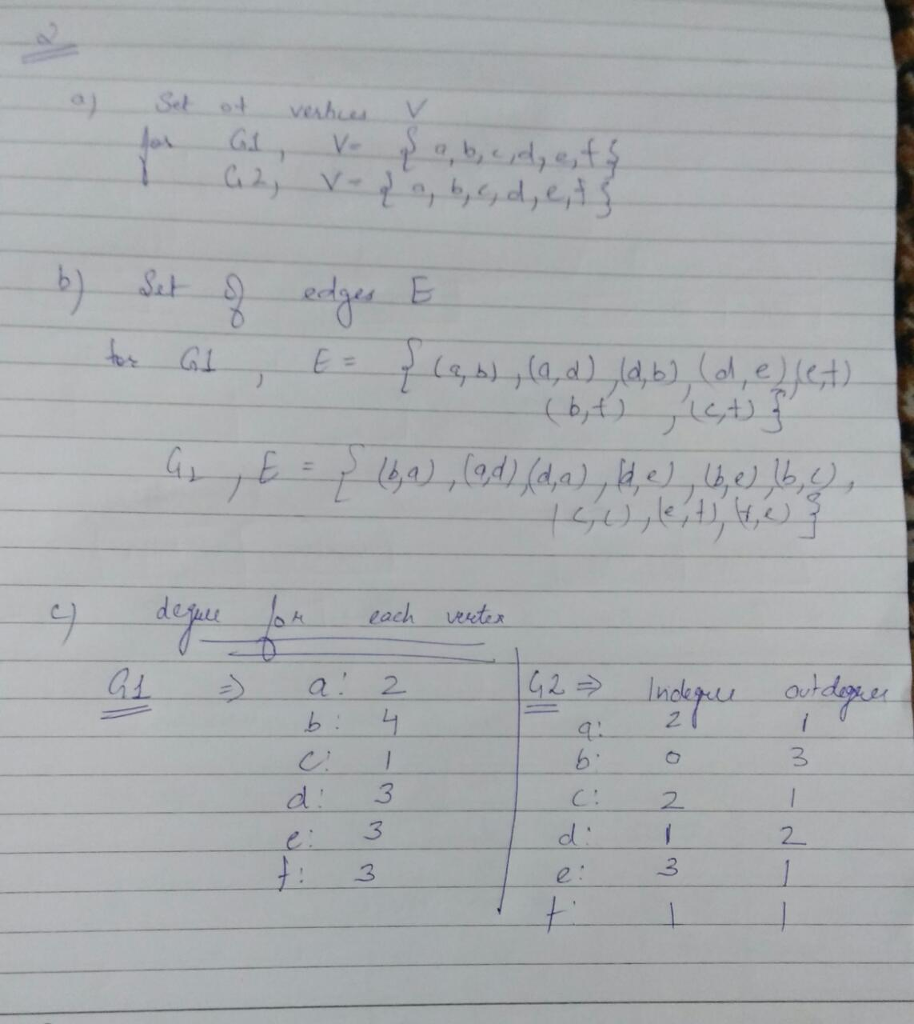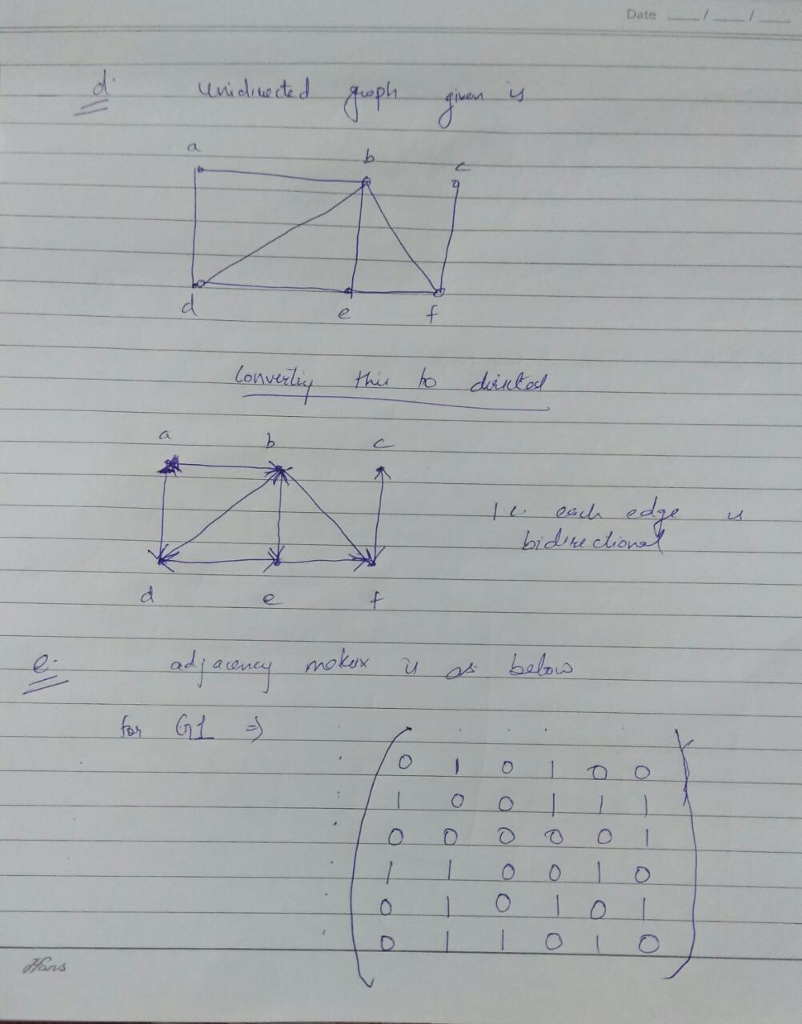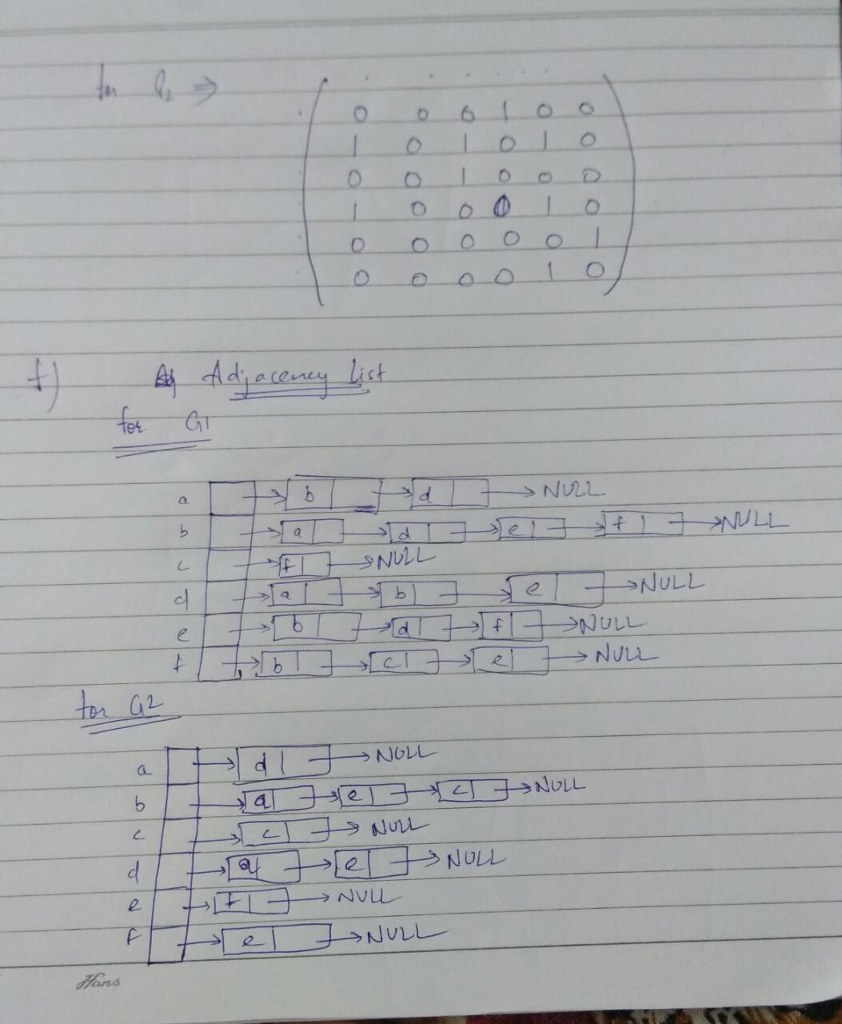# Question & Answer: Graph Terminology and Special Types of Graphs Problem 6 (page 689) For the graph G_1: (a) Specify the set of vertices V. (b) Specify the set of edges E…..

Question 2Graph Terminology and Special Types of Graphs Problem 6 (page 689) For the graph G_1: (a) Specify the set of vertices V. (b) Specify the set of edges E. (c) Give the degree for each vertex (d) Draw the directed graph that can be used to represent this undirected graph (e) Give the adjacency matrix representation for this graph. (Assume vertices are sorted lexicographically) (f) Give the adjacency list representation for this graph.